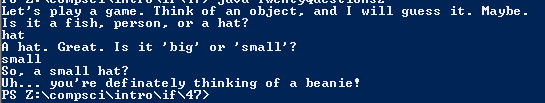# Assignment 47; TwentyQuestions2

## Code

```    // Donovan Rich
// Period 6
// Two More Questions
// TwentyQuestions
// 10/30/2015

import java.util.Scanner;

public class TwentyQuestions2 {

public static void main( String[] args ) {

Scanner keyboard = new Scanner(System.in);

String ans = "N/A", type = "N/A", size = "N/A", guess = "N/A";
boolean big = true;

System.out.println( "Let's play a game. Think of an object, and I will guess it. Maybe." );
System.out.println( "Is it a fish, person, or a hat?" );
ans = keyboard.next();

if ( ans.equals( "fish" ) )
{
type = "fish";
}
if ( ans.equals( "person" ) )
{
type = "person";
}
if ( ans.equals( "hat" ) )
{
type = "hat";
}

System.out.println( "A " + type + ". Great. Is it 'big' or 'small'?" );
ans = keyboard.next();

if ( ans.equals( "big" ) )
{
big = true;
size = "big";
}
if ( ans.equals( "small" ) )
{
big = false;
size = "small";
}

if ( type.equals( "fish" )  && ( big == true ) )
{
guess = "a great white shark";
}
if ( type.equals( "fish"  ) && ( big == false ) )
{
guess = "an anchovy";
}
if ( type.equals( "person"  ) && ( big == true ) )
{
guess = "Chief Bromden";
}
if ( type.equals( "person"  ) && ( big == false ) )
{
guess = "Peter Dinklage";
}
if ( type.equals( "hat" )  && ( big == true ) )
{
guess = "a sombrero";
}
if ( type.equals( "hat" )  && ( big == false ) )
{
guess = "a beanie";
}

System.out.println( "So, a " + size + " " + type + "?" );
System.out.println( "Uh... you're definately thinking of " + guess + "!" );
}
}

```

### Picture of the output